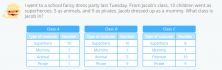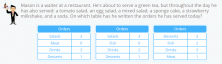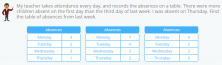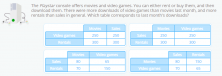Accelerate your child’s learning
Smartick is a fun way to learn math!Oct29

# Math Problems with Data Tables

For today´s entry, we are going to solve math problems where the relevant data is displayed on a table.

The reason is clear – to be able to resolve any situation we need to know how to read, discriminate, and manage all types of data and the ways of exhibiting them, including:

• In text.
• Visual schemes.
• All types of graphics.
• Data Tables.

The following math problems are ordered in such a way to familiarize you, little by little, with different types of tables and ways of using them.

With these exercises, we will learn to identify, based on the statements, the table that represents the data it gives us.

### Math problems where you need to identify the correct table

• #### We are going to begin with examples that imply only single-entry tables

These are the tables that only represent one data characteristic.

For example, a table where you put the birth dates of all your classmates.  This table would show the names of your classmates and their birth dates and only one piece of data would be displayed for each student: ”date of birth.”Identify the table that represents the data listed in the text (Number to number).Identify the table that represents the data mentioned in the text (Words to numbers).Identify the table that represents the data you can interpret from the text (Clues to numbers).
• #### Now we are going to see math problems that use double-entry data tables

These are the tables that represent two data characteristics for each entry.

For example, a table with all the students where, in addition to their birth dates, their number of siblings is listed as well. Two pieces of data are given for each student ”date of birth” and ”number of siblings.”Between different types of double-entry tables, identify which one interprets the text completely (Clues to numbers).

I hope that this series of math problems has helped you learn the basic representation of data in single and double entry data tables. If you would like to continue practicing with these and other types of tables, go to Smartick and try our method.